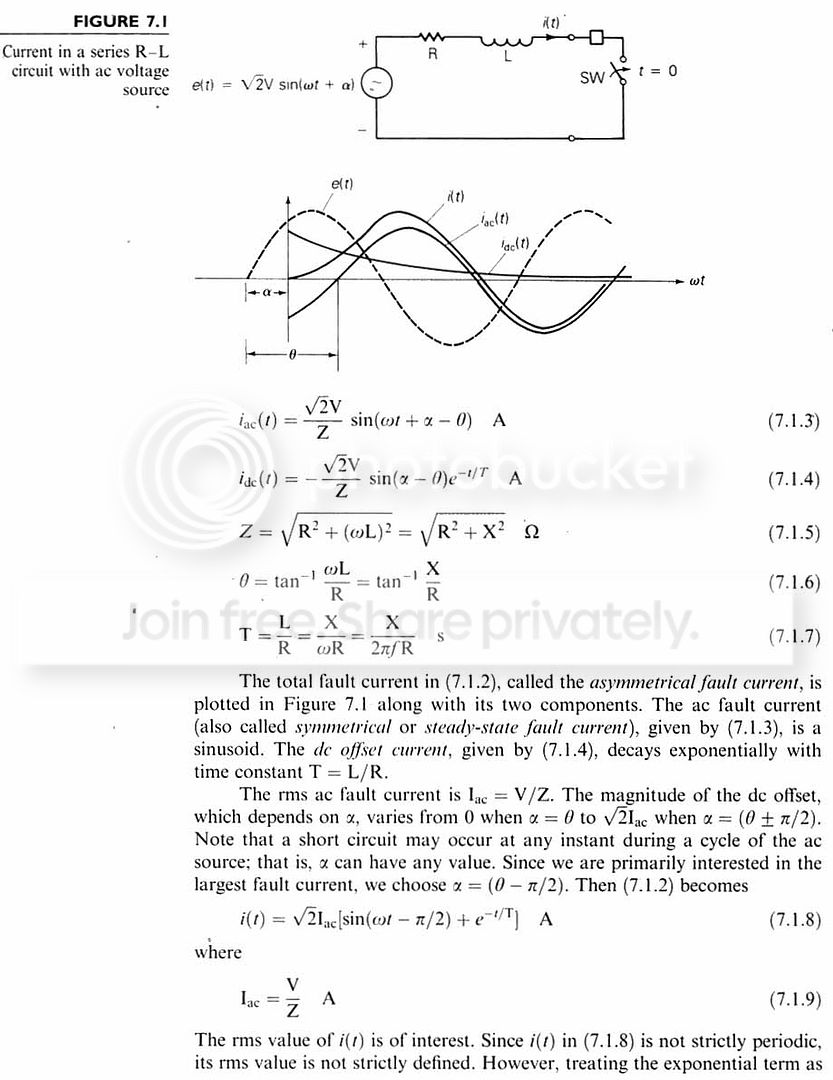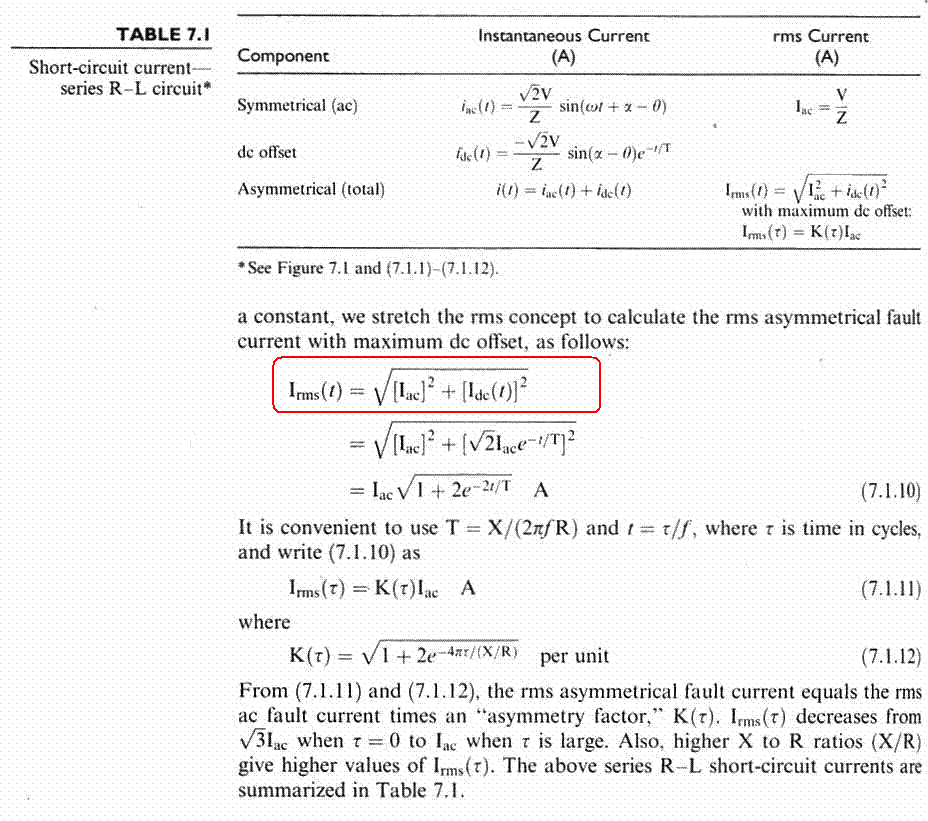# Does a AC circuit have 'instantaneous' RMS current?

Status
Not open for further replies.

#### powersysinstantaneous rms

I don't understand a formula to calculate 'RMS' current stated in "Power System Analysis and Design" by Glover and Sarma. As highlighted by RED color circle in the attached images, pls note that it's Irms(t), not Irms. Therefore, I call it as 'instantaneous' RMS current. Pls correct me if I'm wrong. I don't understand how the red-circled part derived... any idea? Pls advise.

Thanks...#### kalyanramHi Powersys,

Forget everything about the notation used in the problem as its a bit ambiguous. Firstly you do agree that the current as a function of time can be represented in the is harmonics using the Fourier Series then you can say that Irms(t) = (∑(all the coeff)²)½ here you can say a0 is the dc coefficient and the rest are ac hence the result follows.

~Kalyan

#### powersyskalyanram said:
Hi Powersys,

Forget everything about the notation used in the problem as its a bit ambiguous. Firstly you do agree that the current as a function of time can be represented in the is harmonics using the Fourier Series then you can say that Irms(t) = (∑(all the coeff)²)½ here you can say a0 is the dc coefficient and the rest are ac hence the result follows.

~Kalyan
I thought Fourier Series is only applied for periodic signal. But the i(t) is strictly not periodic... Pls correct if I'm wrong... Thanks.

#### kalyanramHi Powersys,
The idea is to look at the dc component as periodic signal with periodicity of T as T tends to infiinity and then go ahead with the rms evaluation. This is a standard method used for extending the fourier series notation to non-periodic signals.

I hope I'm clear this time, let me know in case of any ambiguity.

~Kalyan.

#### miGs

##### Junior Member level 3The encircled formula is the Fault Current taking into account the DC component. Its been simplified by getting the symmetrical fault current then multiplying it with a factor as can be seen from your attachments. The current is still RMS but its the ASYMMETRICAL fault current. Don't confuse it with the "RMS" current.

Status
Not open for further replies.